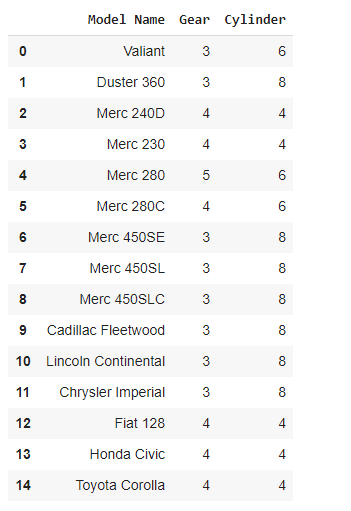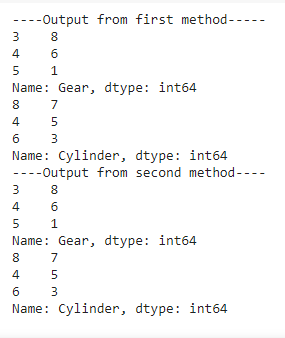# Count unique values with Pandas per groups

• Last Updated : 29 Jul, 2021

Prerequisites: Pandas

In this article, we are finding and counting the unique values present in the group/column with Pandas. Unique values are the distinct values that occur only once in the dataset or the first occurrences of duplicate values counted as unique values.

### Approach:

• Import the pandas library.
• Import or create dataframe using DataFrame() function in which pass the data as a parameter on which you want to create dataframe, let it be named as “df”, or for importing dataset use pandas.read_csv() function in which pass the path and name of the dataset.
• Select the column in which you want to check or count the unique values.
• For finding unique values we are using unique() function provided by pandas and stored it in a variable, let named as ‘unique_values’.

Syntax: pandas.unique(df(column_name)) or df[‘column_name’].unique()

• It will give the unique values present in that group/column.
• For counting the number of unique values, we have to first initialize the variable let named as ‘count’ as 0, then have to run the for loop for ‘unique_values’ and count the number of times loop runs and increment the value of ‘count’ by 1
• Then print the ‘count’, this stored value is the number of unique values present in that particular group/column.
• For finding the number of times the unique value is repeating in the particular column we are using value_counts() function provided by Pandas.

Syntax: pandas.value_counts(df[‘column_name’] or df[‘column_name’].value_counts()

• This will give the number of times each unique values is repeating in that particular column.

For a better understanding of the topic. Let’s take some examples and implement the functions as discussed above in the approach.

Example 1: Creating DataFrame using pandas library.

## Python

 `# importing library``import` `pandas as pd` `# storing the data of cars in the dictionary``car_data ``=` `{``'Model Name'``: [``'Valiant'``,``                           ``'Duster 360'``,``                           ``'Merc 240D'``,``                           ``'Merc 230'``,``                           ``'Merc 280'``,``                           ``'Merc 280C'``,``                           ``'Merc 450SE'``,``                           ``'Merc 450SL'``,``                           ``'Merc 450SLC'``,``                           ``'Cadillac Fleetwood'``,``                           ``'Lincoln Continental'``,``                           ``'Chrysler Imperial'``,``                           ``'Fiat 128'``,``                           ``'Honda Civic'``,``                           ``'Toyota Corolla'``],``            ` `            ``'Gear'``: [``3``, ``3``, ``4``, ``4``, ``5``, ``4``, ``3``, ``3``,``                     ``3``, ``3``, ``3``, ``3``, ``4``, ``4``, ``4``],``            ` `            ``'Cylinder'``: [``6``, ``8``, ``4``, ``4``, ``6``, ``6``, ``8``,``                         ``8``, ``8``, ``8``, ``8``, ``8``, ``4``, ``4``, ``4``]}` `# creating DataFrame for the data using``# pandas DataFrame function.``car_df ``=` `pd.DataFrame(car_data)` `# printing the dataframe``car_df`

Output:Example 2: Printing Unique values present in the per groups.

## Python

 `# importing libraries``import` `pandas as pd` `# storing the data of cars in the dictionary``car_data ``=` `{``'Model Name'``: [``'Valiant'``,``                           ``'Duster 360'``,``                           ``'Merc 240D'``,``                           ``'Merc 230'``,``                           ``'Merc 280'``,``                           ``'Merc 280C'``,``                           ``'Merc 450SE'``,``                           ``'Merc 450SL'``,``                           ``'Merc 450SLC'``,``                           ``'Cadillac Fleetwood'``,``                           ``'Lincoln Continental'``,``                           ``'Chrysler Imperial'``,``                           ``'Fiat 128'``,``                           ``'Honda Civic'``,``                           ``'Toyota Corolla'``],``            ` `            ``'Gear'``: [``3``, ``3``, ``4``, ``4``, ``5``, ``4``, ``3``, ``3``,``                     ``3``, ``3``, ``3``, ``3``, ``4``, ``4``, ``4``],``            ` `            ``'Cylinder'``: [``6``, ``8``, ``4``, ``4``, ``6``, ``6``, ``8``,``                         ``8``, ``8``, ``8``, ``8``, ``8``, ``4``, ``4``, ``4``]}` `# creating DataFrame for the data using pandas``car_df ``=` `pd.DataFrame(car_data)` `# printing the unique values present in the Gear column``# finding unique values present``# in the Gear column using unique() function``print``(f``"Unique values present in Gear column are: {car_df['Gear'].unique()}"``)` `# printing the unique values present``# in the Cylinder column``# finding unique values present in the``# Cylinder column using unique() function``print``(f``"Unique values present in Cylinder column are: {car_df['Cylinder'].unique()}"``)`

Output:From the above output image, we can observe that we are getting three unique value from both of the groups.

Example 3: Another way of finding unique values present in per groups.

## Python

 `# importing libraries``import` `pandas as pd` `# storing the data of cars in the dictionary``car_data ``=` `{``'Model Name'``: [``'Valiant'``,``                           ``'Duster 360'``,``                           ``'Merc 240D'``,``                           ``'Merc 230'``,``                           ``'Merc 280'``,``                           ``'Merc 280C'``,``                           ``'Merc 450SE'``,``                           ``'Merc 450SL'``,``                           ``'Merc 450SLC'``,``                           ``'Cadillac Fleetwood'``,``                           ``'Lincoln Continental'``,``                           ``'Chrysler Imperial'``,``                           ``'Fiat 128'``,``                           ``'Honda Civic'``,``                           ``'Toyota Corolla'``],``            ` `            ``'Gear'``: [``3``, ``3``, ``4``, ``4``, ``5``, ``4``, ``3``, ``3``,``                     ``3``, ``3``, ``3``, ``3``, ``4``, ``4``, ``4``],``            ` `            ``'Cylinder'``: [``6``, ``8``, ``4``, ``4``, ``6``, ``6``, ``8``, ``8``,``                         ``8``, ``8``, ``8``, ``8``, ``4``, ``4``, ``4``]}` `# creating DataFrame for the data using pandas``car_df ``=` `pd.DataFrame(car_data)` `# finding unique values present in the``# groups using unique() function``unique_gear ``=` `pd.unique(car_df.Gear)``unique_cyl ``=` `pd.unique(car_df.Cylinder)` `# printing the unique values present in the Gear column``print``(f``"Unique values present in Gear column are: {unique_gear}"``)` `# printing the unique values present in the Cylinder column``print``(f``"Unique values present in Cylinder column are: {unique_cyl}"``)`

Output:The output is similar but the difference is that in this example we had founded the unique values present in per groups by using pd.unique() function in which we had passed our dataframe column.

Example 4: Counting the number of times each unique value is repeating.

## Python

 `# importing libraries``import` `pandas as pd` `# storing the data of cars in the dictionary``car_data ``=` `{``'Model Name'``: [``'Valiant'``,``                           ``'Duster 360'``,``                           ``'Merc 240D'``,``                           ``'Merc 230'``,``                           ``'Merc 280'``,``                           ``'Merc 280C'``,``                           ``'Merc 450SE'``,``                           ``'Merc 450SL'``,``                           ``'Merc 450SLC'``,``                           ``'Cadillac Fleetwood'``,``                           ``'Lincoln Continental'``,``                           ``'Chrysler Imperial'``,``                           ``'Fiat 128'``,``                           ``'Honda Civic'``,``                           ``'Toyota Corolla'``],``            ` `            ``'Gear'``: [``3``, ``3``, ``4``, ``4``, ``5``, ``4``, ``3``,``                     ``3``, ``3``, ``3``, ``3``, ``3``, ``4``, ``4``, ``4``],``            ` `            ``'Cylinder'``: [``6``, ``8``, ``4``, ``4``, ``6``, ``6``, ``8``,``                         ``8``, ``8``, ``8``, ``8``, ``8``, ``4``, ``4``, ``4``]}` `# creating DataFrame for the data using pandas``car_df ``=` `pd.DataFrame(car_data)` `# counting number of times each unique values``# present in the particular group using``# value_counts() function``gear_count ``=` `pd.value_counts(car_df.Gear)``cyl_count ``=` `pd.value_counts(car_df.Cylinder)` `# another way of obtaining the same output``g_count ``=` `car_df[``'Gear'``].value_counts()``cy_count ``=` `car_df[``'Cylinder'``].value_counts()``print``(``'----Output from first method-----'``)` `# printing number of times each unique``# values present in the particular group``print``(gear_count)``print``(cyl_count)` `# printing output from the second method``print``(``'----Output from second method----'``)``print``(g_count)``print``(cy_count)`

Output:From the above output image, we are getting the same result from both of the methods of writing the code.

We can observe that in Gear column we are getting unique values 3,4 and 5 which are repeating 8,6 and 1 time respectively whereas in Cylinder column we are getting unique values 8,4 and 6 which are repeating 7,5 and 3 times respectively.

Example 5: Counting number of unique values present in the group.

## Python

 `# importing libraries``import` `pandas as pd` `# storing the data of cars in the dictionary``car_data ``=` `{``'Model Name'``: [``'Valiant'``,``                           ``'Duster 360'``,``                           ``'Merc 240D'``,``                           ``'Merc 230'``,``                           ``'Merc 280'``,``                           ``'Merc 280C'``,``                           ``'Merc 450SE'``,``                           ``'Merc 450SL'``,``                           ``'Merc 450SLC'``,``                           ``'Cadillac Fleetwood'``,``                           ``'Lincoln Continental'``,``                           ``'Chrysler Imperial'``,``                           ``'Fiat 128'``,``                           ``'Honda Civic'``,``                           ``'Toyota Corolla'``],``            ` `            ``'Gear'``: [``3``, ``3``, ``4``, ``4``, ``5``, ``4``, ``3``, ``3``,``                     ``3``, ``3``, ``3``, ``3``, ``4``, ``4``, ``4``],``            ` `            ``'Cylinder'``: [``6``, ``8``, ``4``, ``4``, ``6``, ``6``, ``8``,``                         ``8``, ``8``, ``8``, ``8``, ``8``, ``4``, ``4``, ``4``]}` `# creating DataFrame for the data using pandas``car_df ``=` `pd.DataFrame(car_data)` `# finding unique values present in the particular group.``name_count ``=` `pd.unique(car_df[``'Model Name'``])``gear_count ``=` `pd.unique(car_df.Gear)``cyl_count ``=` `pd.unique(car_df.Cylinder)` `# initializing variable to 0 for counting``name_unique ``=` `0``gear_unique ``=` `0``cyl_unique ``=` `0` `# writing separate for loop of each groups``for` `item ``in` `name_count:``    ``name_unique ``+``=` `1` `for` `item ``in` `gear_count:``    ``gear_unique ``+``=` `1` `for` `item ``in` `gear_count:``    ``cyl_unique ``+``=` `1` `# printing the number of unique values present in each group``print``(f``'Number of unique values present in Model Name: {name_unique}'``)``print``(f``'Number of unique values present in Gear: {gear_unique}'``)``print``(f``'Number of unique values present in Cylinder: {cyl_unique}'``)`

Output:From the above output image, we can observe that we are getting 15,3 and 3 unique values present in Model Name, Gear and Cylinder columns respectively.

My Personal Notes arrow_drop_up